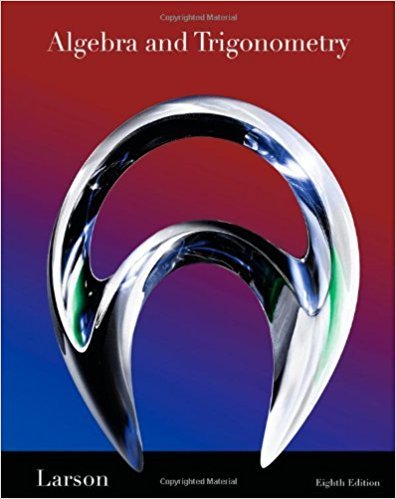×
×

# Solutions for Chapter 8.5: Trigonometric Form of A Complex Number## Full solutions for Algebra and Trigonometry | 8th Edition

ISBN: 9781439048474Solutions for Chapter 8.5: Trigonometric Form of A Complex Number

Solutions for Chapter 8.5
4 5 0 299 Reviews
14
3
##### ISBN: 9781439048474

Chapter 8.5: Trigonometric Form of A Complex Number includes 116 full step-by-step solutions. This textbook survival guide was created for the textbook: Algebra and Trigonometry, edition: 8. This expansive textbook survival guide covers the following chapters and their solutions. Since 116 problems in chapter 8.5: Trigonometric Form of A Complex Number have been answered, more than 51626 students have viewed full step-by-step solutions from this chapter. Algebra and Trigonometry was written by and is associated to the ISBN: 9781439048474.

Key Math Terms and definitions covered in this textbook
• Back substitution.

Upper triangular systems are solved in reverse order Xn to Xl.

• Block matrix.

A matrix can be partitioned into matrix blocks, by cuts between rows and/or between columns. Block multiplication ofAB is allowed if the block shapes permit.

• Characteristic equation det(A - AI) = O.

The n roots are the eigenvalues of A.

• Commuting matrices AB = BA.

If diagonalizable, they share n eigenvectors.

• Complex conjugate

z = a - ib for any complex number z = a + ib. Then zz = Iz12.

• Cyclic shift

S. Permutation with S21 = 1, S32 = 1, ... , finally SIn = 1. Its eigenvalues are the nth roots e2lrik/n of 1; eigenvectors are columns of the Fourier matrix F.

• Echelon matrix U.

The first nonzero entry (the pivot) in each row comes in a later column than the pivot in the previous row. All zero rows come last.

• Eigenvalue A and eigenvector x.

Ax = AX with x#-O so det(A - AI) = o.

• Elimination matrix = Elementary matrix Eij.

The identity matrix with an extra -eij in the i, j entry (i #- j). Then Eij A subtracts eij times row j of A from row i.

• Free columns of A.

Columns without pivots; these are combinations of earlier columns.

• Identity matrix I (or In).

Diagonal entries = 1, off-diagonal entries = 0.

• Least squares solution X.

The vector x that minimizes the error lie 112 solves AT Ax = ATb. Then e = b - Ax is orthogonal to all columns of A.

• Left inverse A+.

If A has full column rank n, then A+ = (AT A)-I AT has A+ A = In.

• Linear transformation T.

Each vector V in the input space transforms to T (v) in the output space, and linearity requires T(cv + dw) = c T(v) + d T(w). Examples: Matrix multiplication A v, differentiation and integration in function space.

• Matrix multiplication AB.

The i, j entry of AB is (row i of A)·(column j of B) = L aikbkj. By columns: Column j of AB = A times column j of B. By rows: row i of A multiplies B. Columns times rows: AB = sum of (column k)(row k). All these equivalent definitions come from the rule that A B times x equals A times B x .

• Network.

A directed graph that has constants Cl, ... , Cm associated with the edges.

• Positive definite matrix A.

Symmetric matrix with positive eigenvalues and positive pivots. Definition: x T Ax > 0 unless x = O. Then A = LDLT with diag(D» O.

• Projection p = a(aTblaTa) onto the line through a.

P = aaT laTa has rank l.

• Skew-symmetric matrix K.

The transpose is -K, since Kij = -Kji. Eigenvalues are pure imaginary, eigenvectors are orthogonal, eKt is an orthogonal matrix.

• Vector space V.

Set of vectors such that all combinations cv + d w remain within V. Eight required rules are given in Section 3.1 for scalars c, d and vectors v, w.

×